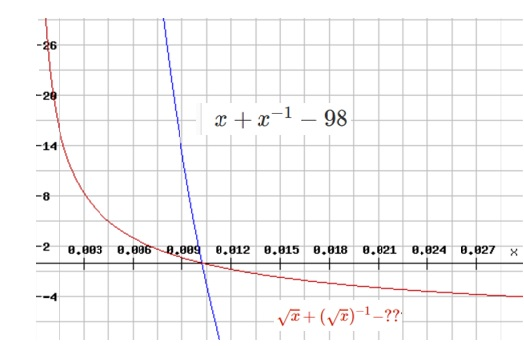# $x + x^{-1} = 98$

Algebra Level 2

If $x$ satisfies the equation $x + x^{-1} =98$, what is the value of $\sqrt x + (\sqrt x)^{-1}$?×